﻿ Innovative Mnemonics Make Chemical Education Time Economic – A Pedagogical Review ArticlePublications are Open
Access in this journal
Article Versions
Export Article
• Normal Style
• MLA Style
• APA Style
• Chicago Style
Research Article
Open Access Peer-reviewed

Innovative Mnemonics Make Chemical Education Time Economic – A Pedagogical Review Article

Arijit Das
World Journal of Chemical Education. 2018, 6(4), 154-174. DOI: 10.12691/wjce-6-4-2
Received August 02, 2018; Revised September 05, 2018; Accepted September 25, 2018

Abstract

In this review article, formulae based on innovative mnemonics have been discussed to create interest and remove phobia of students in the field of chemical education. Educators can use these numerous mnemonics in their teaching style in the classroom lectures after discussing conventional methods to make chemistry intriguing. Here, I have tried to focus some time economic mnemonics by including thirty-three (33) new formulae in the field of chemical education. It will encourage students to solve multiple choice type questions (MCQs) at different competitive examinations in a time economic ground. This review article emphasizes chemical education in the light of a variety of mnemonic techniques to make it metabolic, time economic and intriguing for students because the use of mnemonics in classroom lectures is an essential tool to become a distinguished educator.

1. Introduction

The conventional methods for determination of hybridization of simple molecules including heterocyclic compounds, bond order of diatomic species having (1-20)e-s using M.O.T., bond-order of oxide-based acid radicals, prediction of spin state using spin multiplicity value, aromatic and anti-aromatic behavior of organic compounds including heterocyclic compounds, evaluation of magnetic behaviour of diatomic species having (1-20)e-s with M.O.T., calculation of number bonds in olefinic hydrocarbons and alkynes etc. is time consuming 1, 2, 3, 4, 5, 6, 7, 8, 9, 10, 11, 12, 13, 14, 15, 16, 17, 18, 19.

Keeping this in mind, in this pedagogical review article, I have introduced some innovative mnemonic techniques to make chemistry metabolic, time economic and interesting 20, 21, 22, 23, 24, 25, 26, 27. Here, I have tried to discuss them abruptly.

2. Methodology

2.1. Innovative Mnemonics for Predicting Hybridization State of Simple Molecules or Ions
Hybridization state theory

Prof. Pauling (1931), first developed the Hybridization state theory in order to explain the structure of molecules such as methane (CH4) using atomic orbitals 1, 2. This concept was developed for simple chemical systems but this one applied more widely later on and from today’s point of view, it is considered an operative empirical for excusing the structures of organic and inorganic compounds along with their related problems.

Conventional method for prediction of hybridization state

Hybridization state for a molecule can be calculated by the formula 0.5 (V+H−C+A), Where, V = Number of valance electrons in central atom, H = Number of surrounding monovalent atoms, C = Cationic charge, A = Anionic charge.

2.1.1. Prediction of sp, sp2, sp3 Hybridization State

Hybridization is nothing but the mixing of orbital’s in different ratio and the newly mixed orbitals called hybrid orbitals. The mixing pattern is as follows:

s + p (1:1) - sp hybrid orbital;

s + p (1:2) - sp2 hybrid orbital ;

s + p (1:3) - sp3 hybrid orbital

Formula: prediction of sp, sp2, and sp3 hybridization state

Power on the Hybridization state of the central atom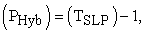where, PHyb = Power on the Hybridization state of the central atom,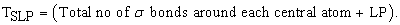From the Lewis structure of a molecule, first of all, predict the number of sigma bonds (σ-bonds), pi bonds (π-bonds) and the lone pair of electrons (LP) if any. All single (-) bonds are the σ bond, in the double bond (=) there is 1σ and 1π, in triple bond (≡) there is 1σ and 2π (exclude π bond). In addition to these each Co-ordinate bond (→) can be treated as 1σ bond. This formula is applicable up to four (04) TSLP. If the power of the hybridization state (PHyb) will be 03, 02 and 01 then the hybridization state will be sp3, sp2 and sp respectively 21, 24, 26.

2.1.2. Prediction of sp3d, sp3d2, sp3d3 Hybridization State

In case of sp3d, sp3d2 and sp3d3 hybridization state there is a common term sp3 for which four (04) TSLP is responsible. So, with four (04) TSLP, for each additional TSLP (additional sigma bond or lone pair of electron), added one d orbital gradually as follows:-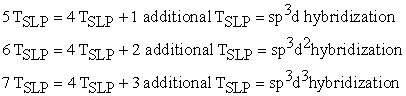In case of cationic species, requisite electron/electrons must be removed from the outermost orbit of the central atom and in case of anionic species, added requisite electron with the outermost electrons of the central atom 21, 24, 26, 29, 30.

2.2. Innovative Mnemonic for Predicting Hybridization State of Hetero Atom in Different Heterocyclic Compounds
Classification of Lone Pair of Electrons in heterocyclic compounds

Lone Pair of electrons can be generally classified into two types as Delocalized lone pair of electron (DLP)

and Localized lone pair of electron (LLP) as follows:

i)Delocalized lone pair of electron (DLP): When lone pair of electron of hetero atom undergoes delocalization through conjugation then it is to be treated as delocalized lone pair of electron (DLP).

Hetero atom (atom containing lone pair of electron) which is directly attached with single bonds only from all ends is to be considered as DLP containing hetero atom and its lone pair is to be treated as (DLP).

Eg. In Pyrrole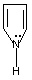lone pair of N atom is to be treated as DLP because it is directly attached with three single bonds only.

ii) Localized lone pair of electron (LLP): When lone pair of electron of hetero atom does not undergo delocalization through conjugation then it is to be treated as Localized lone pair of electron (LLP). Hetero atom (atom containing lone pair of electron) which is directly attached with single and double bonds with the ring system is to be considered as LLP containing hetero atom and its lone pair is to be treated as localized lone pair of electron (LLP).

Eg. In Pyridine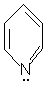lone pair of N atom is to be treated as LLP because it is directly attached with double and single bonds with the ring system.

Planarity of Heterocyclic Compounds

Planarity is one of the vital features for prediction aromatic, anti aromatic and non aromatic behavior of heterocyclic compounds or other organic compounds. For aromatic and anti aromatic behavior the compound must be planar, whereas, non planar compound is non aromatic in nature 12, 13, 14, 15, 16, 17, 18. Planarity of heterocyclic compounds depends on the nature of the hybridization state of carbon and hetero atoms present in it. When all atoms (carbon and hetero) in the heterocyclic compounds having sp2 hybridized then it is planar but when there is a mixing of sp2 and sp3 hybridization state then it is treated as non planar.

2.2.1. Prediction of sp2 and sp3 Hybridization State

Formula: prediction of sp2 and sp3 hybridization state

Power on the Hybridization state of the hetero atom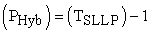where, PHyb = Power on the Hybridization state of the hetero atom, TSLLP = (Total no of σ bonds around each central atom + LLP), LLP = Localized lone pair of electron.

If the power of the hybridization state (PHyb) will be 03, 02 and 01 then the hybridization state will be sp3, sp2 and sp respectively. All single (-) bonds are σ bond, in double bond (=) there is one σ and one π. In addition to these each localized lone pair of electron (LLP) can be treated as one σ bond 27.

2.3. Predicting the Bond-Order of Diatomic Species

Bond-order usually predicted from the Molecular Orbital Theory. Molecular Orbital Theory (M.O.T.) was first proposed by Friedrich Hund and Robert Mulliken in 1933 3, 4. They developed an approach to the covalent bond formation which is based upon the effects of the various electron fields upon each other and which employs molecular orbital rather than atomic orbital. Each such orbital characterizing the molecule as a whole is described by a definite combination of quantum numbers and possesses relative energy value.

Conventional method for prediction of bond order with molecular orbital theory:

Increasing energetic order of different molecular orbitals for number of electrons < or = 14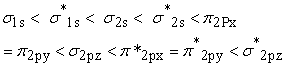Increasing energetic order of different molecular orbitals for number of electrons > 14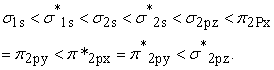In this energetic order, there is five bonding molecular orbitals (BMOs), σ1s, σ2s, π2px, π2py, σ2pz and five anti bonding molecular orbitals (ABMOs), σ*1s, σ*2s, π*2px, π*2py, σ*2pz.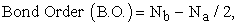where Nb = Number of electrons in bonding molecular orbitals, Na = Number of electrons in anti bonding molecular orbitals.

First of all, classify the molecules or ions having (1-20)e-s into the following four (4) types based on total number of electrons present in them 21, 24, 25, 26, 29, 30.

2.3.1. Molecules and Ions Having Total no of Electrons within the Range (2-6):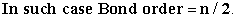2.3.2. Molecules and Ions Having Total no of Electrons within the Range (2-6):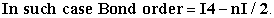2.3.3. Molecules and Ions Having Total no of Electrons within the Range (6-14):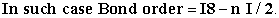2.3.4. Molecules and Ions Having Total no of Electrons within the Range (14-20):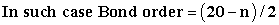[Where n = Total no of electrons, ‘I I’ indicates Mod function i.e. the value of bond order is always positive].

2.4. Prediction of Bond-Order of Oxide Based Acid Radicals

Bond order of oxide based acid radicals can be calculated from the simple molecular formulae of the acid radicals in the following way 21, 24, 26, 29, 30.

In case of oxide based acid radicals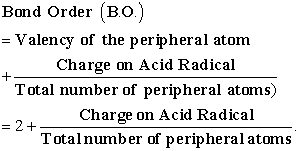2.4. Prediction Magnetic Behavior of Diatomic Species

The present study involves three new formulae by just manipulating the number of unpaired electrons (n) using mod function (based on Applied Mathematics) and by means of these n values one can easily stumble the magnetic moment values in Bohr-Magneton using spin only formula μs = √n(n+2) B.M., where B.M. = Bohr Magneton = unit of magnetic moment, n = number of unpaired electrons 21, 24, 25, 26, 29, 30.

First of all we classify the molecules or ions depending on the total number of electrons present in them in the following three (03) sets.

Set-1: Molecules or ions having (1-3)e-s, (3-5)e-s, (5-7)e-s, (7-10)e-s, (13-16)e-s

Set-2: Molecules or ions having (10-13)e-s and (16-19)e-s

Set-3: Molecules or ions having 20 e-s

Then for different set we have to use three different formulae to calculate the number of unpaired electrons and thus magnetic moment (μs in B.M.) can be evaluated in the following way:

2.5.1. F-1(For Set-1) - for the determination of number of unpaired electrons (n) of molecules or ions having total number of electrons (1-3), (3-5), (5-7), (7-10) and (13-16)e-s:

In this case, the number of unpaired electrons n = [I (ND - total e-s) I].

Here, ND = next digit i.e. digit next to minimum digit and ‘I I’ indicates mod function.

Eg:Molecules or ions having (1-3)e-s, in this case ND = 2 because here minimum digit is 1.

For the molecules or ions containing (3-5)e-s, (5-7)e-s, (7-10)e-s, and (13-16)e-s the ND value will be 4, 6, 8 and 14 respectively.

Hence, the value of n = [I (4-total e-s) I ]; [ I (6- total e-s) I ] [ I (8- total e-s) I] and [I (14- total e-s) I ] respectively.

2.5.2. F-2 (For Set-2) - for the determination of number of unpaired electrons (n) of molecules or ions having total number of electrons (10-13) and (16-19):

In this case, the number of unpaired electrons n = [I (PD - total e-s) I].

Here, PD = Penultimate electron digit (i.e. before last electron).

For the molecules or ions containing (10-13) and (16-19)e-s the PD value will be 12 and 18 respectively.

Hence, the value of n = [I (12 - total e-s) I] and [I (18 - total e-s) I ] respectively.

2.5.3. F-3 (For Set-3) - for the determination of number of unpaired electrons (n) of molecules or ions having total number of electrons 20:

In this case, the number of unpaired electrons n = [(20 - total e-s)].

2.6. Evaluating Spin Multiplicity

Spin-multiplicity value and its corresponding spin state first discovered by Friedrich Hund in 1925. The formula which is generally used for the prediction of spin multiplicity value is [(2S+1), where S = Σs = total spin quantum no] is time-consuming 6. To keep the matter in mind a simple innovative method has to be introduced for calculation of spin-multiplicity value and thus its corresponding spin state in the easiest way by ignoring the calculation of total spin quantum number (S = Σs).

First of all, we should classify the species (atoms, molecules, ions or complexes) for which spin multiplicity value should be evaluated into three types based on the nature of alignment of unpaired electrons present in them 21, 24, 26, 29, 30.

2.6.1. Species Having Unpaired Electrons in Upward Alignment (↑):

In this case, spin multiplicity = (n+1); where n = number of unpaired electrons

2.6.2. Species Having Unpaired Electrons in Downward Alignment (↓):

In this case spin multiplicity = (-n+1); Here (-ve) sign indicate downward arrow.

2.6.3. Species Having Unpaired Electrons in both Mixed Alignment (↑)(↓) :

In this case spin multiplicity = [(+n) + (-n) + 1]; where, n = number of unpaired electrons in each alignment. Here, (+ve) sign and (–ve) sign indicate upward and downward alignment respectively.

2.7. Innovative Mnemonics for Identifying Aromatic and Anti-Aromatic Organic Compounds

It was first devised by Huckel in 1931 12, 13, 14, 15, 16, 17, 18. The present study will be an innovative method involving two formulae by just manipulating the number of π bonds within the ring system and delocalized electron pair (excluding π electron pair within the ring system) with one (01) 20, 21, 24.

Conventional methods:

Aromatic nature of organic compound

1. Cyclic molecule,

2. Planar molecule in which all bonded atoms lie in same plane (having sp2 hybridized)

3. Conjugated molecule with conjugated π-electron system,

4. Contains (4n + 2) π electrons, where, n is a positive integer (n = 0,1,2,3 etc.)

Anti Aromatic nature of organic Compound:

1. Cyclic molecule,

2. Planer molecule in which all bonded atoms lie in same plane (having sp2 hybridized)

3. Conjugated molecule with conjugated π-electron system,

4. 4nπ electrons, where, n is a positive integer (n = 0,1,2,3 etc.)

Non Aromatic Nature of organic Compound:

If a compound violates any one of the above three conditions (1 or 2 or 3) then it is non aromatic in nature.

2.7.1. Prediction of Aromatic Behavior

In the first case, the compound must be cyclic, planar (i.e. all the carbon atoms having same state of hybridization) and conjugated with even number of A value, where [A = πb+e-p+1(constant)], here πb = number of π bonds within the ring system and e-p = number of electron pair outside or adjacent to the ring system i.e. ring contains hetero atom (atom contains lone pair of electrons) which can undergo delocalization and each negative charge if present may be treated as one pair of electrons.

If the value of ‘A’, for a certain organic compound comes out as an even number then this compound will be treated as an aromatic compound.

2.7.2. Prediction of Anti-aromatic Behavior

In the second case, the compound must be cyclic, planar (i.e. all the carbon atoms having same state of hybridization) and conjugated with odd number of A value, where [A = πb+e-p+1(constant)], here πb = number of π bonds within the ring system and e-p = number of electron pair outside or adjacent to the ring system i.e. ring contains heteroatom which can undergo delocalization and each negative charge if present, may be treated as one pair of electrons.

If the value of ‘A’, for a certain organic compound comes out as an odd number then this compound will treat as an anti-aromatic compound.

2.7.3. General Condition for Non-aromatic behavior of Organic Compounds

Any compound that lacks one or more of the above features i.e. it may be acyclic / non-planar, is to be treated as non aromatic. But in this case, ‘A’ value may be even or odd number.

It is always to be noted that if the ring contains hetero atom like N, O, S etc, in this case, we must count that electron pair in the evaluation of ‘A’ value which can undergo delocalization. We never count localized electron pair.

2.7. Innovative Mnemonics for the Prediction of Aromatic, Anti Aromatic behavior of Heterocyclic

Compounds with DLP:

The present study will be an innovative mnemonic involving calculation of ‘A’ value by just manipulating the no of π bonds within the ring system and delocalized lone pair of electron (DLP) with one (01) 27.

The heterocyclic compound having cyclic, planar, conjugated (i.e. all the carbon atoms having the same state of hybridization, sp2) with an even number of ‘A’ value will be treated as aromatic in nature and with an odd number of ‘A’ value will be treated as anti aromatic in nature.

Formula: Evaluation of A Value to predict Aromatic and Anti Aromatic Nature

A = πb+DLP+1(constant) = even no = Aromatic

A = πb+DLP+1(constant) = odd no = Anti Aromatic

where, πb = number of π bonds with in the ring system;

DLP = Delocalized lone pair of electron.

In case of a multi hetero atom based heterocyclic compound, containing both DLP and LLP hetero atoms,

Aromatic and Anti Aromatic behavior should be predicted with respect to DLP based hetero atom only.

But when heterocyclic compounds contain both LLP based hetero atoms then Aromaticity should be predicted with respect to that hetero atom which contains lowest possible position number as per IUPAC nomenclature or any one of the hetero atom.

2.9. Calculating of π-bonds, σ-bonds, single and double bonds in Straight Chain and Cycloalkene Systems:

The molecular formula which defines a very large number of chemical structure, in this particular case, it is a herculean task to calculate the nature and number of bonds. Earlier Badertscher et al studied a novel formalism to characterize the degree of unsaturation of organic molecules 19.

But no such work has not been taken till now to calculate the number and types of bonds in open chain olefinic system having complex molecular formulae like C176H250, C2000H2000.

Keeping this in view, a rapid innovative method has been proposed for the calculation of the number of π-bonds, σ-bonds, single and double bonds with the help of following 06 (six) completely new formulae for certain aliphatic unsaturated open-chain and cyclic olefinic hydrocarbons 21, 23, 24.

2.9.1. For Open Chain Aliphatic Hydrocarbons

(i) Calculation of π-bonds and double bonds (P):

The number of π bonds or double bonds for a straight chain olefin is P= [(2X-Y)/2] + 1; Where, X = number of carbon atoms; Y = number of hydrogen atoms and P = number of π bonds/double bonds.

(ii) Calculation of σ-bonds (S):

The number of σ bonds for a straight chain olefin is S = [X + Y - 1]; where, X = number of carbon atoms; Y = number of hydrogen atoms and S = number of sigma bonds (σ-bonds).

(iii) Calculation of Single bonds (A):

The total number of single bond for a straight chain olefin is A = [(3Y/2)-2]; where A = number of single bonds and Y is number of hydrogen atoms.

2.9.2. For Cyclic Aliphatic Olefinic Hydrocarbons

(i) Calculation of π-bonds and double bonds (Pc):

The number of π bonds or double bonds for an aliphatic cyclic olefin is Pc= [(2X-Y)/2]; Where, X = number of carbon atoms; Y = number of hydrogen atoms and Pc = number of π bonds or double bonds in the cyclic olefinic system.

(ii) Calculation of σ-bonds (Sc):

The number of σ bonds for an aliphatic cyclic olefin is Sc = [X + Y]; Where, X = number of carbon atoms; Y = number of hydrogen atoms and Sc = number of sigma bonds (σ-bonds) in cyclic olefinic system.

(iii) Calculation of Single bonds (Ac):

The total number of single bonds in aliphatic cyclic olefin can be calculated by using the formula Ac = [3Y/2]; where Ac = number of single bonds and y is number of hydrogen atoms in aliphatic cyclic olefin.

2.10. Calculation of π-bonds, σ-bonds, Single and TRIPLE BOnds in Straight Chain Alkyne and Cycloalkyne Systems

The number and types of bonds in open chain and cycloalkynes having the complex molecular formula is a Herculean task. Keeping this in view, a rapid innovative method has been proposed for the calculation of number of π-bonds, σ-bonds, single and triple bonds with the help of following 08 (eight) completely new formulae by just manipulating the number of carbon and hydrogen atoms by using some factors for certain aliphatic unsaturated open chain and cycloalkynes 21, 22, 24.

2.10.1. Open Chain Aliphatic Alkynes

(i) Calculation of π-bonds (P):

The number of π bonds for an aliphatic open chain alkyne, where there is one or more than one triple bonds is P= [{(2X-Y)/2} + 1]; where, X = number of carbon atoms; Y = number of hydrogen atoms and P = number of π bonds.

(ii) Calculation of σ-bonds (S):

The number of σ bonds for an aliphatic open chain alkyne, where there is one or more than one triple bonds is S= [X+Y-1]; where, X = number of carbon atoms; Y = number of hydrogen atoms and S = number of σ bonds.

(iii) Calculation of Single bonds (A):

The total number of single bond for an aliphatic open chain alkyne, where there is one or more than one triple bonds is A = [{(2X+5Y)/2} - 3]/2, where, A = number of single bonds, X = number of carbon atoms and Y = number of hydrogen atoms.

(iv) Calculation of Triple bonds (T):

In the first case, we have to count the number of carbon atoms (X) and the number of hydrogen atoms (Y) in a given unsaturated hydrocarbon containing triple bonds. The formula to calculate the number of triple bonds for an aliphatic open chain alkyne, where there is one or more than one triple bonds is T= [{(2X-Y)/2} + 1]/2; where, X = number of carbon atoms; Y = number of hydrogen atoms and T = number of triple bonds.

2.10.2. Cycloalkynes

(i) Calculation of π-bonds (Pc):

In the first case, we have to count the number of carbon atoms (X) and the number of hydrogen atoms (Y) in the given unsaturated cycloalkyne. The formula to calculate the number of π bonds for an aliphatic cycloalkyne is Pc= [(2X-Y)/2]; where, X = number of carbon atoms; Y = number of hydrogen atoms and Pc = number of π bonds in the cycloalkyne system.

(ii) Calculation of σ-bonds (Sc):

The number of σ bonds for an aliphatic cycloalkyne is Sc = [X + Y]; where, X = number of carbon atoms; Y = number of hydrogen atoms and Sc = number of sigma bonds (σ-bonds) in cycloalkyne system.

(iii) Calculation of Single bonds (Ac):

The total number of single bond for an aliphatic cyclo alkyne is Ac = [{(2X+5Y)/2}]/2; where, Ac = number of single bonds in cycloalkyne, X = number of carbon atoms and Y = number of hydrogen atoms.

(iv) Calculation of Triple bonds (T):

The number of triple bond is Tc= [{(2X-Y)/2}]/2; where, X = number of carbon atoms; Y = number of hydrogen atoms and Tc = number of triple bond.

3. Results and Discussion

Prediction of the hybridization state (sp, sp2 & sp3) of simple molecules and ions can be well explained in the following way

Eg.:

a. NH3: In NH3, central atom N is surrounded by three N-H single bonds i.e. three (03) sigma (σ) bonds and one (01) lone pair (LP). So, TSLP = 4, in NH3, hence, power on the hybridization state of N in NH3, (PHyb) = (TSLP) – 1= (3+1)-1 = 3 i.e. hybridization state = sp3.

b. H2O: In H2O, central atom O is surrounded by two O-H single bonds i.e. two (02) sigma (σ) bonds and two (02) lone pairs. So, in this case, power on the hybridization state of O, (PHyb) = (TSLP) – 1= = (2+2)-1 = 3, i.e. hybridization state of O in H2O = sp3.

c. H3BO3:- In H3BO3, B has (Figure 1), three (03) σ bonds only (no LPs) and oxygen has two (02) σ bonds and two (02) lone pair of electrons, so, in this case, power on the hybridization state of B, (PHyb) = (TSLP) – 1= (3+0)-1 = 2 i.e. B is sp2 hybridized in H3BO3. On the other hand, the power of the hybridization state of O, (PHyb) = (TSLP) – 1= = (2+2)-1= 3 i.e. hybridization state of O in H3BO3 is sp3.

d. I-Cl: In I-Cl, I and Cl both have one (01) σ bond and three (03) lone pair of electrons, so, in this case, power on the hybridization state of both I and Cl, (PHyb) = (TSLP) – 1= (1+3) - 1 = 3 i.e. hybridization state of I and Cl both are sp3.

e.CH2 = CH2: In C2H4, each carbon (Figure 1), is attached with two (02) C-H single bonds (2 σ bonds) and one C=C bond (1σ bond), so, altogether there are 3 sigma bonds. So, in this case, the power on the hybridization state of both C, (PHyb) = (TSLP) – 1= (3+0)-1 = 2 i.e. hybridization state of both carbons are sp2.

• Figure 1. Structure of H3BO3 and C2H4

f. O3: Ozone (O3) exists as a stable form of cyclic ozone (Figure 2) and its structure is equilateral triangle 2, 5. In which each centre O atom has two (02) O-O single bonds (2σ bonds) and two (02) lone pair of electrons. So, in this case, power on the hybridization state of central O atom (PHyb) = (TSLP) – 1= (2+2) - 1 = 3 i.e. hybridization state of centre atom O in cyclic O3 is sp3.

But the resonance description of ozone involves two structures (Figure 3), in which, central oxygen atom of ozone will have sp2 hybridization state. In this case, the central O atom has two (02) σ bonds and one (01) lone pair of electron (LP = 01), hence, power on the hybridization state of central O atom in resonance hybrid of ozone, (PHyb) = (TSLP) – 1= (2+1) - 1 = 2 (sp2).

• Figure 2. Equilateral triangle structure of cyclic ozone (O3)
• Figure 3. Resonating structures of Ozone (O3)

g. S8: The ordinary form of sulfur (orthorhombic sulfur, yellow crystals) contains octatomic molecules (S8), in which, S can form single covalent bonds with two other S atoms in a zigzag fashion (Figure 4), into a long chain. In this case, each sulfur atom attached with two (02) adjacent σ bonds and two (02) lone pair of electrons (LP = 2). Hence, power on the hybridization state of any S atom (PHyb) = (TSLP) – 1= (2+2) - 1 = 3 i.e. hybridization state of S atoms in S8 is sp3.

h.P4: In P4, the four P atoms are arranged at the corners of a regular tetrahedron (Figure 4). Here, each P atom forms three covalent bonds (3σ bonds) and one lone pair of electron (LP = 1). Hence, power on the hybridization state of any P atom (PHyb) = (TSLP) – 1= (3+1) - 1 = 3 i.e. hybridization state of P atoms in P4 is sp3.

• Figure 4. Zigzag structure of S8 and Tetrahedron structure of P4

i. CO32- : In the valence bond structure of carbonate ion (CO32-), the central carbon atom does not contain any lone pair of electron (LP = 0) but it has three (03) σ bonds (Figure 5). Hence, power on the hybridization state of central C atom in carbonate ion, (PHyb) = (TSLP) – 1= (3+0) - 1 = 2 (sp2). But in resonance hybrid of CO32- (Figure 6), carbon atoms are in sp2 hybridization state due to 3 σ bonds and no lone pair of electrons (LP = 0).

• Figure 5. Valence bond structure of carbonate ion (CO32-)
• Figure 6. Resonance hybrid of CO32-

Prediction of the hybridization state (sp3d, sp3d2 & sp3d3) of simple molecules and ions can be well explained in the following way

Eg:-

a. I3-: In Tri iodide ion (I3-), central I atom has 2σ bonds and 3 lone pair of electrons (LP = 3) (Figure 7). Hence for central I, there is 5 TSLP So, 5 TSLP = 4 TSLP + 1 additional TSLP = sp3d hybridization.

• Figure 7. Linear structure of tri iodide ion (I3-)
• Figure 8. Structure of IF4+and XeF4

b. IF4+: In IF4+ (Figure 8), I have 7 e-s in its outermost shell, so, in this case, subtract one e- from 7 i.e. 7 – 1 = 6. So, out of 6 electrons, 4 electrons form four (04) I-F σ bonds and there is one (01) LP. So, altogether there are 5 TSLP. So, 5 TSLP = 4 TSLP + 1 additional TSLP = sp3d hybridization.

• Figure 9. Regular / Normal Molecular Geometry without Lone pair of electrons
• Figure 10. Subnormal Molecular Geometry with Lone pair of electrons

c. XeF4: In XeF4 (Figure 8), Xe, an inert gas, consider 8 e-s in its outermost shell, four (04) of which form four (04) Xe-F sigma bonds and there are two (02) lone pair of electrons, so, altogether there is 06 TSLP = 4 TSLP + 2 additional TSLP = sp3d2 hybridization.

d. IF7: In IF7, there is seven (07) I-F single bonds i.e. 7σ bonds and no lone pair of electron (LP), so, altogether there is 07 TSLP = 4 TSLP + 3 additional TSLP = sp3d3 hybridization.

In case of determination of the hybridization state by using the above method, one must have a clear idea about the outermost electrons of different family members in the periodic table as follows: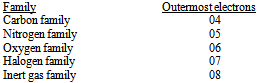The geometry of simple molecules or ions

In absence of lone pair of electrons (LPs) a molecule or ion exhibit regular geometry (Figure 9). For sp, sp2, sp3, sp3d, sp3d2 and sp3d3 hybridization state, geometry will be linear, trigonal planar, tetrahedral, trigonal bipyramid, octahedral and pentagonal bipyramid respectively, whereas for the same hybridization state in presence of the lone pair of electrons they exhibit subnormal geometry (Figure 10) 8, 9, 10.

Prediction of the hybridization state (sp2 & sp3) of hetero atom in heterocyclic compounds can be well explained in the following way

Hybridization state of hetero atom in heterocyclic compounds can be calculated from the total number of σ bonds around hetero atom and number of localized lone pair of electrons (TSLLP) on the hetero atom and subtract one (01) from this total value of TSLLP to get the hybridization state (sp2 & sp3) of the hetero atom in the heterocyclic compounds.

Adequate examples on the prediction of the hybridization state from the corresponding TSLP value (total number of σ bonds around the central atom + lone pair of electron on central atom) of the central atom have been explored in Table 1. Molecular Geometry (normal and subnormal) and bond angle with respect to the corresponding hybridization state and lone pair of electrons of simple molecules or ions have been displayed in Table 2. Hybridization state of hetero atom in heterocyclic compounds containing one, two or more same or different number of hetero atoms with the help of the localized lone pair of electron (LLP) has been evaluated in Table 3.

Prediction of bond order for diatomic species having (1-20)e-s:

The graphical representation (Figure 11), shows that bond-order gradually increases to 1 in the range (0-2) electrons then falls to zero in the range (2-4) electrons then it further rises to 1 for (4-6) electrons and once again falls to zero for (6-8) electrons then again rises to 3 in the range (8-14) electrons and then finally falls to zero for (14-20) electrons. For total no of electrons 2, 6 and 14, one can use multiple formulae, because they fall in the overlapping region in which they intersect with each other.

• Figure 11. Graphical Representation of B.O. with number of electrons

Prediction of bond order for oxide based acid radicals:

It can be illustrated by the following examples

Eg.

ClO4- : (Valency of one Peripheral atom Oxygen = 2, Charge on acid radical = -1, Total Number of Peripheral atoms = 04), Therefore B.O. = 2 + (-1/4) = 1.75

ClO3- : (Valency of one Peripheral atom Oxygen = 2, Charge on acid radical = -1, Total Number of Peripheral atoms = 03), Therefore B.O. = 2 + (-1/3) = 1.66

ClO2- : (Valency of one Peripheral atom Oxygen = 2, Charge on acid radical = -1, Total Number of Peripheral atoms = 02), Therefore B.O. = 2 + (-1/2) = 1.5

AsO43- : (Valency of one Peripheral atom Oxygen = 2, Charge on acid radical = -3, Total Number of Peripheral atoms = 04), Therefore B.O. = 2 + (-3/4) = 1.25

AsO33-: (Valency of one Peripheral atom Oxygen = 2, Charge on acid radical = -3, Total Number of Peripheral atoms = 03), Therefore B.O. = 2 + (-3/3) = 1.0

SO42- : (Valency of Peripheral atom Oxygen = 2, Charge on acid radical = -2, Number of Peripheral atoms = 04), Therefore B.O. = 2 + (-2/4) = 1.5

SO32- : (Valency of Peripheral atom Oxygen = 2, Charge on acid radical = -2, Number of Peripheral atoms = 03), Therefore B.O. = 2 + (-2/3) = 1.33

PO43- ; (Valency of Peripheral atom Oxygen = 2, Charge on acid radical = -3, Number of Peripheral atoms = 04), Therefore B.O. = 2 + (-3/4) = 1.25

BO33- ; (Valency of Peripheral atom Oxygen = 2, Charge on acid radical = -3, Number of Peripheral atoms = 03), Therefore B.O. = 2 + (-3/3) = 1

CO32- ;(Valency of Peripheral atom Oxygen = 2,Charge on acid radical = -2, Number of Peripheral atoms = 03), Therefore B.O. = 2 + (-2/3) = 1.33

SiO44-:(Valency of Peripheral atom Oxygen = 2,Charge on acid radical = - 4, Number of Peripheral atoms = 04), Therefore B.O. = 2 + (- 4/4) = 1

Relation of different parameters (Bond length, Bond Strength, Bond energy, Thermal stability and Reactivity) with Bond order:

B.O. α 1 / Bond length or Bond distance;

B.O. α Bond strength;

B.O. α Bond Energy;

B.O. α Bond dissociation Energy;

B.O. α Thermal Stability; B.O. α 1 / Reactivity

Correlation among / between Literature values of bond-distances (Å) and bond dissociation energy (KJ mol-1) of some oxide based acid radicals with their predicted bond order values:

Literature values of the Cl-O average bond lengths in ClO4-,ClO3- and ClO2- are 1.50, 1.57 and 1.64 (Å) for their predicted bond orders values 1.75, 1.6 and 1.5 respectively ; As-O average bond lengths in AsO43- and AsO33- are 1.75 and 1.77 (Å) for their predicted bond order values 1.25 and 1.0 respectively which suggests that with increasing Bond-Order bond length decreases.

Literature values of bond dissociation energies of O2+, O2 and O2- are respectively 642.9, 493.6 and 395.0 KJ mol-1 for their predicted bond orders values 2.5, 2.0 and 1.5 respectively; bond dissociation energies of NO+, NO and NO- are respectively 1046.9, 826.9 and 487.8 KJ mol-1 for their predicted bond order values 3.0, 2.5 and 2.0 respectively, which suggests that with increasing Bond-Order bond dissociation energy increases.

Magnetic Behavior of Diatomic Species:

Magnetic behavior of diatomic species can be predicted by classifying the diatomic species having the total number of electrons (1-20) into three different sets and thus calculating the number of unpaired electron/electrons (n) by using three different formulae for three different sets.

Bond order of homo and heteronuclear diatomic molecules or ions having total number of electrons fall in the range (1-20) can be evaluated from their total number of electrons only without drawing their electronic configuration and their magnetic moments (μs) in Bohr Magneton (B.M.) can be evaluated by calculating the number of unpaired electrons has been illustrated in Table 4 and Table 5 respectively.

Spin multiplicity and its corresponding spin state:

First of all, we should classify the species (atoms, molecules, ions or complexes) for which spin multiplicity value and its corresponding spin state should be evaluated into three types based on the nature of alignment of unpaired electrons (upward, downward, or mixed alignment) present in them.

For upward alignment

Eg.

Spin multiplicity = (n +1) = (1+1) = 2 (spin state = doublet); (2+1) = 3 (spin state = triplet) and (3 + 1) = 4 (spin state = quartet) respectively.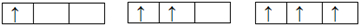Spin multiplicity = (n +1) = (2 + 1) = 3 (in this case ignore paired electrons) (spin state = triplet) and (1 + 1) = 2 (spin state = doublet)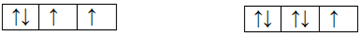Spin multiplicity = (n +1) = (0 + 1) = 1 (spin state = singlet)

For downward alignment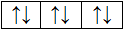Spin multiplicity = (-n +1) = (-1 + 1) = 0; (-2 + 1) = -1 and (-3 + 1) = -2 respectively.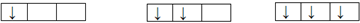Spin multiplicity = (-n + 1) = (-2 + 1) = -1( ignore paired electrons) and (-1 + 1) = 0 respectively.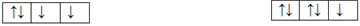For mixed (upward & downward) alignment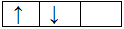Here total no of unpaired electrons = 2 in which one having upward direction (+1) and other having downward mode (-1).

Hence Spin multiplicity = [(+n) + (-n) +1] = [(+1) + (-1) + 1] = 1 (spin state = singlet)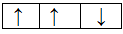Here the total no of unpaired electrons = 3 in which two unpaired electrons lie in upward (+2) and one unpaired electrons lie in downward (-1) .

Hence Spin multiplicity = [(+n) + (-n) + 1] = [(+2) + (-1) + 1] = 2 (spin state = doublet)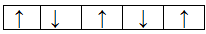Here the total no of unpaired electrons = 5 in which three unpaired electrons lie upward (+3) and two unpaired electrons lie downward (-2).

Hence Spin multiplicity = [(+n) + (-n) + 1] = [(+3) + (-2) +1] = 2 (spin state = doublet)

For 1, 2, 3, 4, 5, 6 or >6 spin multiplicity values (n+1), where n = number of unpaired electrons, the corresponding spin state will be singlet, doublet, triplet, quartet, quintet and multiplet respectively.

Aromatic and Anti-Aromatic nature of Organic Compounds along with their Omission behavior:

If the compound must be cyclic, planar, conjugated with even and odd number of A value, [where, A = πb+e-p+1(constant), here πb = number of π bonds within the ring system and e-p = delocalized lone pair of electrons (DLP) and each negative charge if present may be treated as one pair of electrons] will be aromatic and anti aromatic nature respectively.

Stability / reactivity / acidity of different organic compounds with the help of Aromaticity:

If we easily predict the nature of organic compound i.e. aromatic, anti aromatic or non aromatic then we can resolve different kind of problems regarding stability, reactivity, acidity etc. by using the following supposition.

Order of stability is aromatic > non aromatic > anti aromatic

Order of reactivity is Anti-aromatic > non aromatic > aromatic

Order of Acidity directly proportional to the stability of the Conjugate base

Eg: cyclopentadienyl anion(aromatic) > cyclopentadiene (non-aromatic) > cyclopentadienyl cation (anti aromatic). Hence, cyclopentadiene (its conjugate base i.e. Cyclopentadienyl anion is aromatic in nature) is much more acidic than cycloheptatriene (its conjugate base i.e. Cycloheptatrienyl anion is anti-aromatic in nature).

Omission behavior of aromatic and non aromatic organic compounds:

There are some compounds which do not follow the above rules of A value. Huckel’s also cannot explain the aromatic or non-aromatic behavior of these compounds. These compounds have been represented below.

Cyclodecapentaene (Figure 12), is non aromatic due to the interaction of the hydrogen of 1 and 6, it is non planar, although here, A = πb + DLP + 1(constant) = 5 + 0 + 1 = 6 (even number).

Pyrene (Figure 12), is a polycyclic aromatic hydrocarbon consisting of four fused benzene rings, resulting in a flat aromatic system. It has 8 π bonds and zero (0) DLP. Here, A = 8 + 0 + 1 = 9 (odd number). But still it is aromatic instead of anti aromatic because double bonded C15-C16 does not take part in resonance.

• Figure 12. Geometry of Cyclodecapentaene and Pyrene

Aromatic and Anti-Aromatic behavior of Heterocyclic Compounds along with their omission nature:

The heterocyclic compound having cyclic, planar, conjugated (i.e. all the carbon atoms having the same state of hybridization, sp2) with an even number of ‘A’ value will be treated as aromatic in nature and with an odd number of ‘A’ value will be treated as anti aromatic in nature.

In case of a multi hetero atom based heterocyclic compound, containing both DLP and LLP hetero atoms, Aromatic and Anti Aromatic behavior should be predicted with respect to DLP based hetero atom only.

Eg. Benzothiazole (Figure 13), is a multi hetero atom based heterocyclic compound, containing both DLP and LLP hetero atoms. Here, for N, DLP = 0 , LLP = 1 and for S, DLP = 1, LLP =1, so, in this case ‘A’ value should be calculated with respect to S only not N. Here, A = 4 + 1 + 1 = 6 (even no) = Aromatic.

But when heterocyclic compounds contain both LLP based hetero atoms then Aromaticity should be predicted with respect to that hetero atom which contains lowest possible position number as per IUPAC nomenclature or any one of the hetero atom.

Eg. Imidazole (Figure 13) is a multi hetero atom based hetero cyclic compound in which, N1 is DLP based hetero atom and N3 is LLP based hetero atom. In this case Aromaticity should be predicted with respect to the DLP based hetero atom N1. For N1, A = πb+DLP+1(constant) = 2+1+1 = 4 (even No) - Aromatic

Eg. Pyrimidine (Figure 13) is a multi hetero atom based hetero cyclic compound in which, both N1 & N3 are in same environment based hetero atoms (LLP based hetero atoms). In this case Aromaticity should be predicted with respect to N1 (lowest possible position number as per IUPAC nomenclature).For N1, A = πb+DLP+1(constant) = 3+0+1 = 4 (even no) – Aromatic.

• Figure 13. Structure of Benzothiazole, Imidazole and Pyrimidine

Omission behavior of some heterocyclic compounds with respect to their Aromatic / Anti Aromatic and Non Aromatic nature:

Heterocyclic compounds containing different DLP based hetero atoms (one contains vacant d orbitals) :

In Phenothiazine (Figure 14), there is two DLP based hetero atoms N and S. In between N and S, since S having vacant d orbitals, so, in this case ‘A’ value will be predicted with respect to DLP based S hetero atom which contains vacant d orbitals only. Here, A = πb + DLP + 1(constant) = 6 +1+1 = 8 (even no) = Aromatic.

Heterocyclic compounds containing same DLP based heteroatom having no d orbitals:

Omission behavior of some heterocyclic compounds will be observed (Figure 14), when there, is at least two hetero atoms (same or different) but both the hetero atoms do not have any d orbitals (such as O,N etc.) and they are in DLP based environment in the ring system.

These molecules have been studied with advanced molecular orbital techniques known as ‘ab initio calculations’. Ab initio quantum chemistry methods’ are computational chemistry methods based on quantum chemistry 28.

In the case of 1,2-dioxin, 1,4-dioxin and dibenzo-1,4-dioxin there is DLP based O atoms in all the molecules but still they will be non aromatic due to prevention of significant free electron delocalization (makes non conjugated). The π electrons from the carbon bonds and the lone pair electrons on the oxygen atoms do not overlap to a significant degree due to absence of vacant d orbitals in both O atoms in each case (pπ-dπ overlap is not possible here in conjugation). It makes these molecules non conjugated and thus allows the molecules to become non aromatic instead of aromatic (A value = even No).

In the heterocyclic compounds, where, there is two DLP based N atoms instead of two DLP based O atoms or there is one DLP N atom along with one DLP O atom, the same phenomena of non aromatic behavior will be observed. Because, both N and O atoms do not have any vacant d orbitals, and hence pπ-dπ overlap is not possible here in conjugation.

Heterocyclic compounds containing same DLP based hetero atoms having vacant d orbitals:

1,4-dithiin and 1,2-dithiin heterocyclic compounds (Figure 14) are anti aromatic, here both S atoms, having vacant d orbitals, contain one DLP and one LLP and here both DLP of both S atoms participate in the delocalization. Hence, for the prediction of ‘A’ value, consider both DLP (DLP = 2). Here, A = πb + DLP + 1 (Constant) = 2 + 2 + 1 = 5 (odd No) = Anti Aromatic.

• Figure 14. Structure of phenothiazine, 1,2-dioxin, 1,4-dioxin, dibenzo-1.4-dioxin and 1,2-dithiin and 1,4-dithiin

Aromatic, anti-aromatic and non-aromatic behavior of organic compounds including heterocyclic compounds have been illustrated with adequate number of examples in Table 6 and Table 7 respectively.

Calculation of chemical bonds in Straight Chain and Cycloalkene System:

Chemical bonds (π-bonds, σ-bonds, single and double bonds) in the open chain and cyclic olefinic hydrocarbons having complex molecular formulae like C176H250, C2000H2000 can be calculated without drawing their structures by using different formulae, involving the number of carbon and hydrogen atoms only.

Calculation of chemical bonds (π bonds, σ bonds, single and double bonds) in open chain and cyclic olefinic hydrocarbons without drawing their structures have been illustrated in Table 8 and Table 9 respectively.

Calculation of chemical bonds in Straight Chain and Cycloalkyne System:

Chemical bonds (π-bonds, σ-bonds, single and triple bonds) in the open chain alkynes and cycloalkynes can be calculated in the same way by using different formulae, involving the number of carbon and hydrogen atoms without drawing their structure as follows.

E.g.: In cycloheptyne (C7H10), X =7, Y = 10, therefore, number of π bonds (Pc) = (2x7-10)/2 = 2; number of σ bonds (Sc) = (7+10) = 17; numbers of single bonds (Ac) = [{(2X+5Y)/2}]/2 = [{(2x7+5x10)/2}]/2=32/2 = 16 and number of triple bonds (Tc) = [{(2X-Y)/2}]/2 = [{(2 x 7 – 10)/2}]/2 = 2/2 = 1.

Calculation of chemical bonds (π bonds, σ bonds, single and triple bonds) in open chain alkynes without drawing their structures have been illustrated in Table 10.

4. Conclusion

It may be expected that these time economic innovative mnemonics would go a long way to help to the students of chemistry at Undergraduate, Senior Undergraduate and Post-Graduate level who would choose the subject as their career. Experiment in vitro on 100 students showed that by using these formulae students can save up to 30-40 mins time in the examination hall. On the basis of this, I can strongly recommend to use these time economic innovative mnemonics in the field of chemical education.

Acknowledgements

I would be grateful to the SERB, DST, New Delhi, Govt. of India, for their financial assistance (Sanction no – SERB / F / 5537 / 2013-14 dated 27/11/2013 and D.O. No. SB / EMEQ - 014 / 2013).

I want to delegate my innovative review article in the field of chemical education to my beloved father Late Anil Ranjan Das, who was also a chemistry teacher and also the founder of my chemistry world (www.arijitchemistryworld.com) and my beloved mother Mrs.Sulekha Das, who has a great inspiration behind my innovational research work.

Furthermore, I want to give my cordial honor and gratitude to the newly elected Honorable Chief Minister of ‘Tripura’, Mr.Biplab Kumar Deb and Honorable Education & Higher Education Minister of Tripura, Mr.Ratan Lal Nath for their constant valuable sustaining mentality to improve our state in all respect.

In addition to these, I give my special accolade to Mr.Sekhar Datta, Senior Journalist, ‘The Telegraph’, Mr. Sanjib Deb, Editor, ‘North East Colours’, English Daily, Agartala, Tripura, Mr. Jayanta Debnath, Managing Director, tripurainfo.com, Agartala, Tripura, Mr.Santanu Chakraborty, Editor, ‘newsupdateoftripura.com’ and Mr. Debajit Chakraborty, Senior Journalist, ‘newsupdateoftripura.com’, for their constant valuable sustaining mentality to carry out my innovational research work in the field of chemical education.

References

  L. Pauling, “The Nature Chemical Bond. Application of Results Obtained from the Quantum Mechanics and from a Theory of Paramagnetic Susceptibility to the Structure of Molecules.” J. Am. Chem. Soc. 1931, 53(4), pp 1367-1400. In article View Article  L. Pauling and P. Pauling “Chemistry”, Freeman International Ed.,1975, p148, pp 163-167. In article  R S. Mulliken, “Spectroscopy, Molecular Orbitals, and Chemical Bonding”. Nobel Lectures, Chemistry 1963-1970, Amsterdam Elsevier Publishing Company, 1972. In article PubMed  George G. Hall, “The Lennard-Jones Paper of 1929 and the foundations of Molecular Orbital Theory”, Advances in Quantum Chemistry. 1991, 22, pp 1-6. In article View Article  James S. Wright “Theoretical Evidence for a Stable form of Cyclic Ozone, and its Chemical Consequences”, Can. J. Chem. 1973, 51, pp 139-146. In article View Article  T. Engel and P. Reid “Physical Chemistry”. Pearson Benjamin-Cummings, 2006, p473, pp 477-479. In article  J.D. Lee, “Concise Inorg.Chem.” Wiley:India and Oxford, 5th ed., 2009, p944, pp 109-112. In article  J.E. Huheey, E.A. Keiter, R.L. Keiter and O.K. Medhi “Inorganic Chemistry”, 4th ed., PEARSON, India, 2012, pp 172-185. In article  B. Douglas, D. Mcdaniel and J. Alexander “Concepts and Models of Inorg. Chem.” Wiley: India, 3rd ed., 2007, p 157, p 38. In article PubMed  F.A. Cotton, G. Wilkinson and P.L. Gaus “Basic Inorg.Chem.” Wiley: India, 3rd ed., 2007, p107, p523, p 111. In article  B.M. Mahan and R.J. Meyers “International Student Edition University Chemistry”, 4th ed., 1998, pp 599-603. In article  I.L. Finar “Organic Chemistry”, I, Pearson, 6th ed., 2004, p1, p532, pp 577-580. In article  I.L. Finar “Organic Chemistry”, II, Pearson, 5th ed., 2002, pp606-637, ISBN: 81-7808-164-4. In article  R.T. Morrison and R.N. Boyd “Organic Chemistry”, Pearson, 7th ed., 1999, p208. In article  T.W. Graham Solomons and C.B. Fryhle, “Organic Chemistry”, Wiley: India 10th ed., 2012, pp 632-639. In article  J.G. Smith, “Organic Chemistry”, 2nd ed., 2008, pp 616-619. In article  Erich Hückel, “Quantentheoretische Beiträge zum Benzolproblem I. Die Elektronenkonfiguration des Benzols und verwandter Verbindungen”, Z.Phys. 1931, 70, 3(4), pp 204-86. In article  J. March “Advanced Organic Chemistry: Reaction, Mechanisms and Structure”, Willy: New York, 3rd ed., 1985, ISBN 0-471-85472-7. In article  M. Badertscher, K. Bischofberger, M. E. Munk and E. Pretsch, “A Novel Formalism To Characterize the Degree of Unsaturation of Organic Molecules”. J.Chem.Inf. Comput. Sci. 2001, 41(4), pp 889-893. In article View Article  PubMed  A. Das, S. Adhikari, B. Paul, R. Sanjeev and V. Jagannadham “A rapid and innovative method for the identification of aromatic and anti-aromatic nature of organic compounds”, World Journal of Chemical Education, 2013, 1(1), pp 6-8. In article  A. Das, R. Sanjeev and V. Jagannadham “Innovative And Time Economic Pedagogical Views In Chemical Education - A Review Article”,World Journal of Chemical Education,2014,2(3), pp 29-38. In article  A. Das, D. Pal, S. Adhikari, B. Paul, R. Sanjeev and V. Jagannadham “Rapid calculation of the number of π-bonds, σ-bonds, single and triple bonds in aliphatic Unsaturated open chain and cycloalkynes”, World Journal of Chemical Education, 2014, 2(1), pp 1-3. In article  A. Das, D. Pal, B. Paul, R. Sanjeev and V. Jagannadham “Rapid calculation of the number of π-bonds, σ-bonds, single and double bonds in aliphatic unsaturated open chain and cyclic olefinic hydrocarbons”, Education in Chemical Science and Technology , Indian Chemical Society, 2014, 2(1), pp 41-46. In article  A. Das and B. Paul, “Time Economic Innovative Pedagogies In Chemical Science - A Review Article”, Education in Chemical Science and Technology, Indian Chemical Society, 2015, 3(1), pp 1-28. In article  A. Das,“Bond-order and Magnetic Behavior of Diatomic Species without Molecular Orbital Theory”,World Journal of Chemical Education, 2017, 5(4), pp 128-131. In article  A. Das, “Chemical Bonding: Time Economic Innovative Pedagogies - A Review Article”, Global Journal of Science Frontier Research Chemistry, 2017, 17, 2(1), pp 1-16. In article  A. Das, “Lone Pair Electron Discriminate Hybridization with Aromatic and Anti Aromatic behavior of Heterocyclic Compounds - Innovative Mnemonics”, World Journal of Chemical Education 2018, 6(2), pp95-101. In article View Article  Levine, Ira N. “Quantum Chemistry”. Englewood Cliffs, New jersey: Prentice Hall. (1991): 455-544. In article PubMed  A. Das, “A Review of Time Economic Innovative Mnemonics in Chemical Education”, International Journal of Physics and Chemistry Education, 2018, 10(1), pp 27-40. In article View Article  A. Das, “Innovative Mnemonics in Chemical Education - A Review Article”, American Journal of Chemistry and Applications, 2018, 5(1), pp 19-32. In articleThis work is licensed under a Creative Commons Attribution 4.0 International License. To view a copy of this license, visit http://creativecommons.org/licenses/by/4.0/